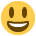#### Howdy, Stranger!

It looks like you're new here. If you want to get involved, click one of these buttons!

Supported by

# [open] JASP: Standard Error in Post-hoc comparisons Repeated Measures ANOVA

edited March 2016

JASP version 0.7.5 Beta 2 running on a Macbook Air
Type of analysis: 2 x 3 repeated measures ANOVA with Post-Hoc comparisons

General comment: This week I've finally started using JASP and I really like it. It makes it much easier to do Bayesian analyses.

This question is related to a standard repeated measures ANOVA in JASP though. I wanted to do the following analysis:

A 2 (Distance: Near, Far) x 3 (Target Modality: A, V, AV) repeated measures ANOVA with Response Time as dependent variable.

There was a significant main effect of Distance, a main effect of Target Modality, and an interaction effect.
Next, I wanted to know whether the levels of Target Modality differed using Post-Hoc comparisons. So I did a Post-Hoc test with just a few clicks.

The confusing part was that the Standard Error of the difference between the levels of Target Modality was exactly the same for all comparisons (A vs. V, A vs. AV, and V vs. AV). I started doubting my self so I did the same analysis in SPSS. There the SE is different for each comparison, resulting in a different outcome of the comparisons. Given that I used the latest Beta version of JASP I wondered whether there is a misunderstanding on my part about calculation of SE of the difference or whether something else is going on in the JASP Beta that isn't supposed to happen.

Best,
Nathan

Table of results in JASP and SPSS:• edited 7:00AM

We use the lsmeans package in R (in combination with the afex package) to calculate post-hoc tests for repeated measure. Here's an example of how we use it:

``````# load packages
require(afex)
require(lsmeans)

# simulate data set
set.seed(1)
dataset <- data.frame(subject = rep(1:20, each = 3),
group = rep (c("A", "B", "C"), 20),
measure = c(rnorm(20, .5), rnorm(20, .6), rnorm(20, .7)))

# fit rm anova using the afex package
model <- aov_car(measure ~ Error(subject/group), data=dataset, type= 3)

# get the least square means
referenceGrid <- lsmeans(model, ~group)

# pairwise comparisons

# reshape to wide format for JASP and SPSS
datasetWide <- reshape(dataset, timevar = "group", idvar = "subject", direction = "wide")

# save data set for analysis in JASP and SPSS
write.csv(datasetWide, "rmDataset.csv")
``````

This code indeed doesn't give the same p-values and SE as SPSS gives. I will contact the author of the afex package next week to see what's happening here. Thanks for your question.

• edited 7:00AM

Ok, thanks for looking into it.
I'm curious to hear what's going on.

• edited 7:00AM

Sorry for not getting back to you sooner. I discussed it with the maintainer of the R package and it appears that the R function assumes that the variances (and thus the SE's) are equal to each other. This makes sense because one of the assumptions in ANOVA is equality of variances. Letting that assumption suddenly go for the post-hoc tests seems like weird behavior from SPSS. I will look into it a bit further to see which method makes more sense (or maybe make an options where you can specify whether you want to assume equal variances or not, but that is gonna take some time).

• edited 7:00AM

Ah, right. I may be wrong, but testing whether the SEs are more or less equal seems different from just setting them as equal. I don't know what's best here, but I would love to hear your thoughts about it when you have time. Thanks for the help so far.

• edited May 2017

I had a similar/related problem with the SEM that comes with the marginal means. Th SEM that JASP reports does not match the SEM that SPSS provides for a between subjects design ANOVA. Why is this

• Hi Bill,
I can have someone look into this, but it would certainly help us if you could provide a specific example so we have some concrete numbers to work with.
Cheers,
E.J.

• Attached is a JASP output of a simple between subjects ANOVA with 2 levels. The SE associated with the marginal means are identical in this output.
The descriptive statistics show that the SDs are markedly different between the two groups, Given that the SE of the mean is the SD/sqrt of n, the SEs with the marginal means cannot possibly be correct. (The SD values given in the descriptive stats check with Excel.)

So why does JASP not give the correct SE value with the marginal means?

• Hi Bill,
Thanks. I will look into it, but I can already tell you that this will most likely be an issue with an R package.
E.J.

• Hi Bill,
You are correct in that the marginal means table produces different standard errors than what you would expect from the descriptives table. JASP uses the "lsmeans" R-package to do the computations for the marginal means table. When there is a balanced ANOVA, the standard errors for estimating the confidence intervals get pooled. Whenever n differs per level of the factor, these standard errors do not get pooled and the marginal means table displays differing standard errors.
In short, the standard errors in the marginal means table are used for testing whether the marginal means differ from 0, whereas the standard deviations reported in the descriptives table are purely descriptive. However, it would be nice to have an additional tickbox that could be unticked to use the unpooled standard errors in these tests - I will discuss this with the programmers and put it on the JASP bucketlistKind regards,
Johnny